# MATLAB Homework Help - We Solve Assignments for You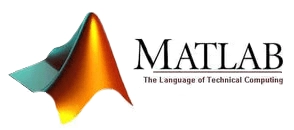Most likely you have one of the two thoughts at the moment: a) “just do my Matlab assignment so that I can forget about it” b) “how can I become good at Matlab and all the related math (do my assignment for now and I will study hard later)”. Or maybe it is a mixture of both… In any way – we can help with Matlab homework and explain the solution (if you have time for that). Usually, our solution code is commented very densely so that you just need to follow with effortless understanding.

Matlab intends to be user-friendly for beginners – it has various graphical user interfaces (GUI) that guide you through the data selection and variable setting process. You might have encountered one by reading in a dataset or using some toolbox. However, these are just the starting steps – you need to learn programming in order to complete your assignments and especially the final Matlab project.

In case you are having issues overcoming Matlab licensing (it is often free/cheap for students, but depends on your university), Octave is a free alternative that uses the same syntax as Matlab. The problem is that Octave does not cover specific functions and most of the toolboxes, just the basics. Nevertheless, we can help with both, your Octave or Matlab problems.

If you have some time, we encourage you to read through the following chapters to get a grasp of what you are dealing with in university overall; if not, just check out a few visually pleasing example solutions way below.

## How Scientists/Engineers Solve Problems in General?

It just happens to be the case that many world situations (from physics to social sciences - anything having some rules of “social” interaction) can be written as a model in mathematics (on some level of abstraction). The same situation might be understood in different ways, using different theories and paying attention to different features. Other than that, most real-life situations are too chaotic or have implicit uncertainty on what state we try to model, so statistics becomes a useful tool.

The different perspectives on the problem are usually taught in different university subjects – you are trained to solve problems in only one way using theories of one subject often together with distinct mathematical tools. There is no single best way to understand the world as there is no single best way to model it – this is why different theories at different scales are useful to solve different problems.

Many rules we create ourselves by technology – social laws, engineering artifacts, or protocols are what restrict how the human world operates, so by knowing the rules we can model it more precisely. All these rules and theories, together with specific mathematical tools help to analyze, model, and find the best path towards the desired outcome in the problem-solving process.

A science or engineering student has a few things to consider during his homework solution process:

• data (a situation, description, and the dataset; rarely data may be asked to collect in active participation),
• theory (extra restrictions, usually in general math formulas),
• correct “tools” (mathematical formalism, that is related to the theories in use),
• problem or expected answer type (requirements, goals, and specifically calculation/process evaluation that your instructor expects you to follow).

Novel combinations of perspective, theory, math, models, etc. are left for a research part, which does not have “assignments” – it is usually a research project under the supervision of a more experienced supervisor. We can help with your Ph.D. or research projects.

Matlab homework usually contains descriptions and vague requirements, while theory to follow and mathematical tools to use are skipped and the student is expected to find them in lecture slides (or more often Google). It causes a lot of confusion for students and when it becomes unbearable - they seek Matlab assignment help.

### Why Matlab?

All the modeling is about computation – you do it mentally or using a computer. Since complex models (having many variables and data) are nearly impossible to build mentally, one needs a mathematical tool, that is flexible, easy to learn, and use in rapid prototyping and solution implementations. Matlab is such a tool and was developed with ease of use in mind. Most general engineering/science tasks that Matlab is used to solve are:

• Difference Equations, Differential Equations: Temporal or spatial rate of change defining equations. Dynamics is the core of real-life modeling.
• Static computations of relations at equilibrium. Getting rid of the time-domain simplifies things a lot (like static mechanics), but ignoring temporal trajectories may oversimplify the situation at hand.
• Statistical modeling, Monte Carlo Methods. Once we have not enough data or circumstances are chaotic - you need to use grouping-based methods. Very rarely such statistical theories converge on actual precise models (as in the case of thermodynamics of gas).
• Symbolic calculations (syms). You often need to develop an analytical solution by hand before implementing a computer program. Matlab has tools to automate error-prone manual symbol manipulations. Easy formula conversion to latex is also a big time saver.

## Matlab Is Popular Among Various University Subjects

Matlab offers many very specific solutions, like bioinformatics or neural network toolboxes – these are examples of problems we rarely have a chance to assist with. These toolboxes cover more or less all the subjects you may study in university. However, most homework tasks are of a more general kind. Because Matlab has both “micro-targeting” toolboxes and great general functionality, it is very popular among our clients.

It is a common practice to give students a task of solving Matlab homework and expect them to integrate the theoretical knowledge from lectures, data analysis, mathematical tools while meeting the requirements in a short period of time. When solving an assignment student is expected to follow exact theories and specific mathematical formalism, but also correctly interpret the task about what the professor really wants. The mathematical solution is usually not so flexible as in verbal analysis in humanities, which means Matlab programming has a high barrier of entry before it produces valuable results.

Below we present the extensive Matlab assignment help list of what you may encounter.

### The Most Popular Matlab Assignments We Help With

As in every programming language, students are first introduced to the logical flow of the program: loops (for, while), user input (input), conditionals (if, else, switch), logical operators (and, or), printing (fprintf); custom functions and objective programming are introduced later. The most popular tasks of the introductory class are:

• printing in a loop, depending on user input, break on condition
• simple probabilistic text games, like 8-Ball (Magic ball), Hangman
• array usage, logical indexing, masking
• manipulation of structure (struct) or cell data types
• exponential growth in bacteria population
• social spreading of virus or social memes among human populations
• introduction to numerical methods: Taylor series, integration, differentiation, differentials approximations: forward, backward, central and other methods; Newton and Bisection Methods for root finding
• prime number finding, recursive Fibonacci numbers, and Pascal triangles, Mandelbrot set (recursive or using for loops), etc.
• simple rocket or “cannonball” projectile (parabola trajectory) or satellite trajectory
• Estimation of ball volume in n-dimensions, using Monte Carlo methods and Euler’s Gamma function

Visually oriented assignments are the most effective in teaching because they make the strongest impression on students, e.g. hypotrochoid curves: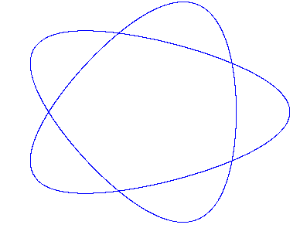Oversimplification (and overspecialization) is often a source of problems in later studies. For example, students are introduced to distance, velocity, acceleration, friction without introducing theory – Newton’s laws of motion expressed in differential equations. Skipping the theory part is a bad practice being already used in schools and universities. Students miss the scientific method overall – active data collection, hypothesis, theory, development of mathematical tools, and the feedback of prediction errors.

### General Data Analysis and Visualization Assignment Help

This kind of Matlab assignments concerns mostly data reading, cleaning, and optimal transformations (for modeling or visualization). Example tasks:

• fopen, Sorting of data, filtering
• plot, plot3, peaks, scatter, stem, etc.
• summation of series using loops
• optimization of some model parameters to fit between input and outputs
• data interpolation and extrapolation, curve fitting using regressions, splines, etc.
• data transformations: Wavelet, Fourier, Hough, PCA, SVD, etc.

Why do we need data transformations? Because correctly done transformation will reduce the number of parameters required to model data accurately; in other words – it reduces the number of unimportant dimensions. For example, in linear projections, linear algebra is used (PCA, SVD): by finding eigenvectors with the highest eigenvalues and discarding other dimensions.

### Ordinary Differential Equations (ODE) Assignment Help: Linear and Non-Linear

Only very simple differential equations have an exact analytical solution. This is why computational models are useful in finding out how differences in time or space generate trajectories and final results. Matlab has in-built ODE solvers, but you might also be asked to work in discrete steps using approximation methods like Euler’s, Runge-Kutta, or finite element method. Other phenomena we usually encounter in non-linear dynamics homework assignments are fixed points, linear stability, numerical solutions, bifurcations, phase oscillations, locking, entrainment, feedback control.

Example Linear differential equation assignments: RC circuit, Harmonic Oscillator, Heat Equation, Maxwell Equation, Schrodinger Equation.
Example Non-linear differential equation assignments: Logistic grow, Pendulum, Forced Oscillator, Lorentz Model, Kuramoto Phase Oscillator, Navier-Stokes (turbulence).

• Crank-Nicolson method for stable thermal conductivity modeling in idealized long cooling copper fin. The result is temperature distribution on the surface of the fin
• heat transfer in 3D body
• membrane and vibrations modeling
• pendulum, double pendulum
• inverse kinematics
• Newton equations in general

There also exist other kinds of differential equations: partial differential equations (PDE), and delayed differential equations (DDE). However, they are rarely taught to undergraduates.

The nice project we have completed using differential equations asked to plot the orbits of the sun, earth, and moon over a specified time period: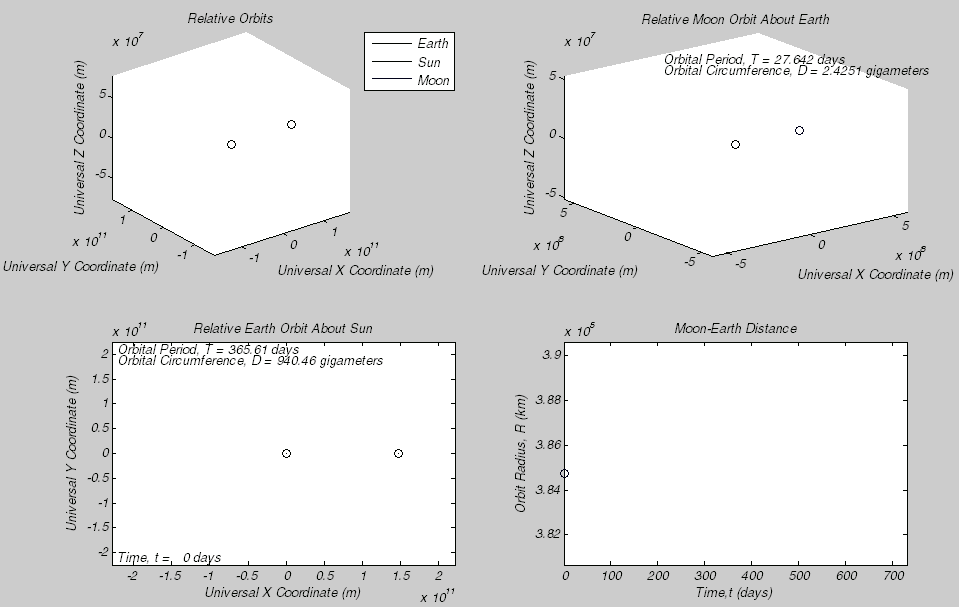Once you are using discrete steps, equations are called difference instead of differential (and functions are called maps). Examples of that are in assignments of chaos, turbulence, logistic map, and game of life:### Image and Video Processing Assignment Help

This group of assignments considers various manipulations and segmentation of an image or video (a stream of images). There are multiple exact engineering solutions built up during the years. Only recently neural network methods started automating these processes. Currently, computer vision is a mixture of old-school well-understood rigid methods and flexible, yet hard-to-train neural networks. Assignments vary from the basic introduction in RGB color channels to complex object detection tasks:

• edit image: erode, blur, convolutions, filter, rotate, flip
• augmented reality (forming the projection matrix from the camera calibration data)
• edge detection and object identification
• region detection
• vehicle license plate recognition, image to text, etc.
• scene rendering, raytracing, shading, etc. Starting with spheres, finishing with realistic faces

### Signal Processing Assignment Help

Signal processing often equivalent to time series analysis, where you try to predict future values, analyze the response of some filter, or detect a pattern (like a steep drop). It covers a very wide array of problems:

• financial time series
• electroencephalography (EEG) heart rate, EEG of brain signals
• psychology or neuroscience students concerned about neuron peristimulus time histogram (PSTH)
• oscillations, resonance, second-order differential equations
• audio signals: gender detection specific features - by pitch, MFCC, cepstrum, etc.### Electrical Engineering and Electronics Assignment Help

• communication engineering: LTS OFDM, BPSK, QAM, Alamouti coding, MIMO systems, the noisy channel, etc.
• physics-oriented calculations: voltage, power, electrical loads, etc.
• electronics signal generators: e.g. square wave generator using sums of sinusoidal signals generates a 1D vector given duration, fundamental frequency, sample rate
• Coding of microchips like Arduino and FPGA

How simple square wave generated using custom code looks like: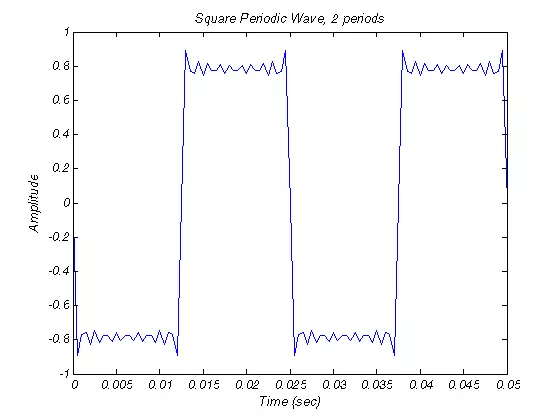### Mechanical Engineering Homework Help

These types of assignments are relatively rare due to the extreme specificity of the tasks.

• static mechanics (bridge or building stability)
• beam theory (tension, compression, bending moment, stress forces, etc.)
• hand calculations and FEA solutions
• A-frame
• LRFD (Load and Resistance Factor Design)
• shear, torsion

### Fluid Calculations Assignment Help

Example question: How long will it take for fluid to leak out of a cylinder with a hole at the bottom? You can proceed in solving it in a few ways: differential equations or “static” math. The most popular fluid-related assignments are exactly like that:

• Navier-Stokes differential equations, turbulence, friction, or frictionless approximation
• Optimal flow distribution in pipes
• Engineering of fluids using the knowledge base, like Moody diagram (considering Raynold’s number). It lets you easily evaluate the turbulence level for pipes for various materials.

We have completed an assignment where the original 1944 diagram replica was made using solely Matlab plotting tools (it took about 7 hours to complete):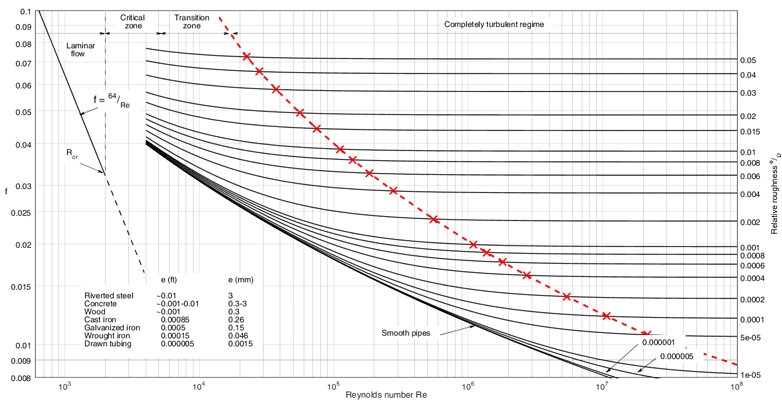### Matlab GUI (guide) and App Designer (appdesigner) Assignment Help

Matlab GUI is a simple to use yet powerful tool to ease human-computer interaction. It can be used to visualize any dialog you would find in any application. Most often GUIs are either very simple used for practice only or very fancy containing lots of code. App designer is a newer shinier version of GUI. Here are some projects that we have provided Matlab GUI homework help with:

• draw something using a mouse and use a neural network to recognize a temporal sequence of dark pixels.
• projectile motion with data entry boxes
• matrix entry and calculation
• shopping item ordering checkout interface
• BlackJack game:Simulink is like another program built inside Matlab. Officially it is yet another toolbox, but a special one. In Simulink, you can build models by connecting visual blocks that each works like a separate system: having its own function to map inputs to outputs.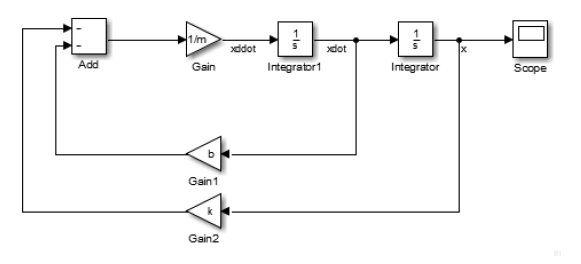Simulink tries to recreate and virtualize regular lab experiences; you even have a data graphing tool that resembles an oscilloscope. It is useful to use when your program code gets too complicated.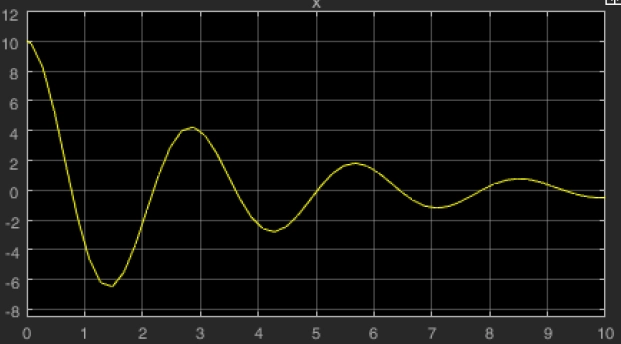## Why do Students Seek Matlab Homework Help?

You need to know theory and math tools in order to interpret the assignment correctly, and you also need to know how to use the provided data and what answers are expected from you. Homework solving should be a process of merging theory, math, data and deepening student’s understanding of the field. However, having too many loose parts in this process ends up in a disaster, which results in excessive stress and avoidance of the subject at all.

Some very best prepared homework assignments contain test cases in compiled function p-files (.p), using which you can check your results. This greatly reduces the uncertainty of the task; however, it requires extra resources from your university.

When poorly prepared assignments, unclear theory lectures, and personal life issues add up, students need help. And receiving help is especially important at home when no university instructor helps you. This is why getting Matlab help online is such a feasible solution!

## Came Here for Matlab Homework Help? Still in Doubt?

We shared what we’ve got with you – this article was possible only because of extensive work we have done during the years of helping students. The process of receiving Matlab homework help is simple:

• Submit order by providing the details (deadline, lecture slides, etc.)
• Wait for a reply email from our expert
• Pay and wait for a solution
• Come to our website to collect the solution

We always encourage students to complete homework on their own, however, it is obvious that in certain circumstances it is not possible – we are always here, 24/7 ready to help.

Is it free to get my assignment evaluated?

Yes. No hidden fees. You pay for the solution only, and all the explanations about how to run it are included in the price. It takes up to 24 hours to get a quote from an expert. In some cases, we can help you faster if an expert is available, but you should always order in advance to avoid the risks. You can place a new order here.

How much does it cost?

The cost depends on many factors: how far away the deadline is, how hard/big the task is, if it is code only or a report, etc. We try to give rough estimates here, but it is just for orientation (in USD):

 Regular homework \$20 - \$150 Advanced homework \$100 - \$300 Group project or a report \$200 - \$500 Mid-term or final project \$200 - \$800 Live exam help \$100 - \$300 Full thesis \$1000 - \$3000

How do I pay?

Credit card or PayPal. You don't need to create/have a Payal account in order to pay by a credit card. Paypal offers you "buyer's protection" in case of any issues.

Why do I need to pay in advance?

We have no way to request money after we send you the solution. PayPal works as a middleman, which protects you in case of any disputes, so you should feel safe paying using PayPal.

Do you do essays?

No, unless it is a data analysis essay or report. This is because essays are very personal and it is easy to see when they are written by another person. This is not the case with math and programming.

Why there are no discounts?

It is because we don't want to lie - in such services no discount can be set in advance because we set the price knowing that there is a discount. For example, if we wanted to ask for \$100, we could tell that the price is \$200 and because you are special, we can do a 50% discount. It is the way all scam websites operate. We set honest prices instead, so there is no need for fake discounts.

Do you do live tutoring?

No, it is simply not how we operate. How often do you meet a great programmer who is also a great speaker? Rarely. It is why we encourage our experts to write down explanations instead of having a live call. It is often enough to get you started - analyzing and running the solutions is a big part of learning.

What happens if I am not satisfied with the solution?

Another expert will review the task, and if your claim is reasonable - we refund the payment and often block the freelancer from our platform. Because we are so harsh with our experts - the ones working with us are very trustworthy to deliver high-quality assignment solutions on time.

Customer Feedback

"Thanks for explanations after the assignment was already completed... Emily is such a nice tutor! "

Order #13073